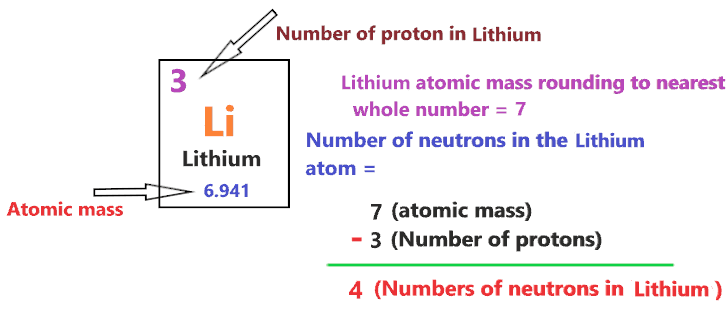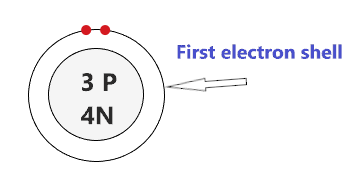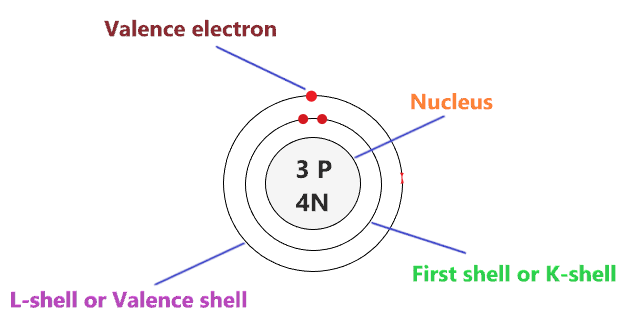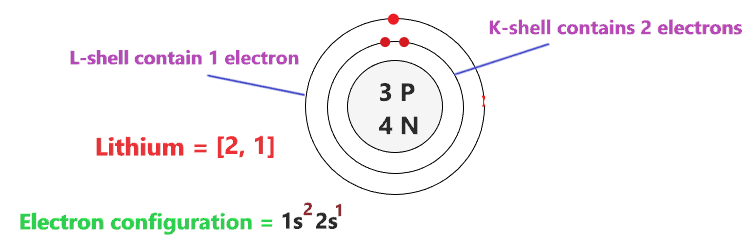# Bohr model of Lithium atom - How to draw Lithium(Li) Bohr-Rutherford diagram?

Home  > Chemistry Article > Lithium Bohr model

The Bohr Model of Lithium(Li) has a nucleus that contains 4 neutrons and 3 protons. This nucleus is surrounded by two-electron shells named K-shell and L-shell. The outermost shell in the Bohr diagram of Lithium contains only 1 electron that also called valence electron.

 Name Lithium Bohr Model Number of neutrons 4 Number of protons 3 Number of electrons 3 Total electron shells 2 Electron in the First shell(K) 2 Electrons in the Second shell(L) 1 Total valence electrons in Lithium 1
Page Contents

## How to draw Bohr Model of Lithium(Li)?

Bohr model describes the visual representation of orbiting electrons around the small nucleus. It used different electron shells such as K, L, M, N…so on. These shells hold a specific number of electrons, the electron shell which is closest to the nucleus has less energy and the electron shell which is farthest from the nucleus has more energy.

Bohr diagram is very interesting and easy to draw. Here, we will draw the Bohr diagram of the Lithium atom with some simple steps.

## Steps to draw the Bohr Model of Lithium atom

1. Find the number of protons, electrons, and neutrons in the Lithium atom

Protons are the positively charged particles and neutrons are the uncharged particles, both these are constituents of the atom nuclei. Electrons are the negatively charged particles that orbit the nucleus of an atom

To find the number of protons an atom contains, just look at their atomic number.

If the atomic number of atom is 13, then proton will also be 13, if atomic number is 14, then proton will also be 14.

So, the atomic number for Lithium is 3, hence, the number of protons in the Lithium atom is also 3.Now, to determine the number of neutrons in an atom, use this formula.

⇒ Number of neutrons in atom = Atomic mass of the atom(rounded to the nearest whole number) – Number of proton in an atom

For example, An atom have 14.998 atomic mass and 8 protons.

Then, to find the number of neutron, round the atomic mass to the near whole number, so, atomic mass 14.998 round to 15.

= (15 – 8 protons) = 7 number of neutrons in the atom

Now, To get the number of neutrons in a Lithium atom, look at its atomic mass which is 6.941 rounded to 7, and the number of protons in Lithium is 3.

∴ Hence, the number of neutrons in Lithium atom = (7 – 3) = 4It should be noted that “The number of electrons in a neutral atom is equal to the number of protons”.

So, the Lithium atom is neutral, hence, its number of electrons will be equal to the number of protons which is three as we already discussed.

⇒ The number of electrons in Lithium atom = 3

⇒ The number of protons in Lithium atom = 3

⇒ The number of neutrons in Lithium atom = 4

2. Draw the nucleus of an atom

A nucleus is a dense and small region that contains the number of protons and neutrons of an atom.

In this step, we have to draw a small circle that consists of a number of protons and the number of neutrons of a Lithium atom.3. Draw the First electron shell

“An electron shell may be thought of as an orbit followed by electrons around an atom’s nucleus.”

The first electron shell is also called the K-shell, this is the closest shell to the nucleus of an atom and can hold a maximum of two electrons.

As we know, Lithium atoms have a total of 3 electrons. So, put two electrons from it, in the first shell, next to each other.We have successfully drawn the first shell of the Lithium atom that can hold only 2 electrons. As Lithium atoms have a total of 3 electrons, and from 3 electrons we have used two electrons in the first shell.

∴ (3 – 2) = 1 electron

Therefore, we are left with only 1 electron, let’s put it in the next shell of the Lithium atom.

4. Draw the Second electron shell

The second shell also called the L-shell that can hold a maximum of 8 electrons. This shell is drawn after the first electron shell.

Since we already used two electrons in the first shell, now, we have to put the remaining 1 electron in the second shell.

In the second electron shell, the electrons are added one at a time, starting from the top position then going in a clockwise direction.

So, we have to put only 1 electron of the Lithium atom in the second shell, hence, just put it at the top position.### Bohr model of the Lithium atom

That’s all, this is our Bohr model of the Lithium atom that contains 3 protons and 4 neutrons in the nucleus region, and 3 electrons are orbited around the nucleus, two electrons in the first shell, and one electron in the second shell.

## Find Valence electron of Lithium through its Bohr diagram

Valence electrons are found in the outermost shell of an atom and they can take participate in the formation of a chemical bond. These electrons have more energy compare to the inner shell electrons.

From the Bohr diagram of an atom, we can easily find the number of valence electrons in an atom by looking at its outermost shell.

So, we have to find a valence electron in the Lithium atom, for this, look at its Bohr diagram.

Bohr’s diagram of Lithium has only two electron shells (K and L), the inner shell is K-shell and the outermost shell is L-shell.

Hence, the electrons found in the L-shell of the Lithium atom are its valence electrons because it is the outermost shell that also called the valence shell.

The L-shell or outer shell of the Lithium Bohr model contains only 1 electron, therefore, the number of valence electrons in the Lithium atom is 1.## Electron dot diagram of the Lithium atom

Electron dot diagram also called lewis structure which represents the valence electrons of atoms.

As, from the Bohr diagram of Lithium, we got to know, it has 1 valence electron.

So, just represent the 1 valence electrons around the Lithium atom as a dot.## The electron configuration of Lithium

Electron configuration is the distribution of electrons of an atom or molecule in atomic or molecule orbitals”

Lithium has an atomic number of 3 and it contains a total number of 3 electrons. From the Bohr model of Lithium, we know, 2 electrons are in the K-shell and 1 electron is in the L-shell.

So, based on the shell, the electronic configuration of the Lithium atom is [2, 1].

Or the electronic configuration of Lithium is 1s22s1 since it contains a total of 3 electrons, the first two-electron will go in 1s orbital and the next one in 2s orbital.

S orbital can hold maximum of 2 electrons.## FAQ

### How many electron shells a Lithium Bohr model contains?

Electron shell also called energy level, you can find the number of electron shells for an element by knowing its period number in the periodic table.

The elements or atoms in the first period of the periodic table have one energy level or one electron shell, same as, the elements in the second period have two energy levels or two-electron shells and so on…

So, the Lithium atom belongs to the 2nd Period in the periodic table, hence, the number of electron shells for the Bohr model of Lithium will be 2 (K-shell and L-shell).

### What is the outer shell of the Bohr diagram of the Lithium atom?

The outermost shell also called the valence shell, which contains valence electrons of the atom.

According to the Bohr diagram of Lithium, the outer shell is L-shell which contains only 1 valence electron.

## Properties of Lithium

1. It appears silvery-white in color.
2. It is one of the lightest metal and solid elements.
3. It is highly reactive and flammable.
4. It has a boiling point of 1330 °C and a melting point of 180.50 °C.
5. It has a body-centered cubic crystal structure.

## Summary

• The Bohr model of Lithium is drawn with only two electron shells, the first shell contains 2 electrons and the second shell contains only 1 electron.
• Lithium is neutral and its atomic number is 3, hence, the number of protons and electrons available for its Bohr diagram is also 3.
• The number of neutrons for the Bohr diagram of Lithium can be found by subtracting the number of protons from the atomic mass(rounded to the nearest whole).
• The electron configuration of Lithium in terms of the shell is [2, 1], or in normal form, it is [He] 2s1.
##### Subscribe to Blog via Email

Join 2 other subscribers

Share it...